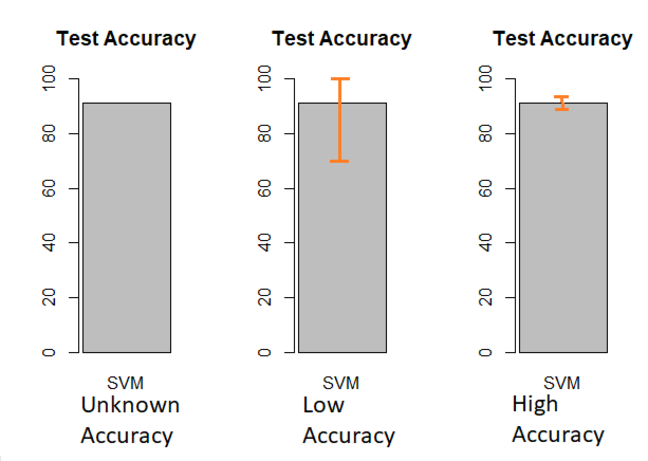## Want to keep learning?

This content is taken from the The Open University & Persontyle's online course, Advanced Machine Learning. Join the course to learn more.
2.10

# Analysis of Data

Perhaps the most common question asked by a student learning about data science and machine learning is how should we divide up data into training, validation and test sets?

If you asked this, chances are that you were told there is no rigorous way of answering the question. Perhaps you were given some arbitrary rule of thumb, or told that it was an ‘art’ rather than a ‘science’. Actually, there are ways of rigorously analysing the division of data, and we will introduce them in this step.

Before doing so, it is worth considering the purpose of the different data sets and the corresponding questions that we want to answer when analyzing the suitability of a division into them.

Data Purpose Question
Training Fit parameters of models Have we sufficient data to fit parameters as well as possible?
Validation Compare relative performance of models in order to select best Have we sufficient data to be able to confidently identify the best model?
Test Estimate expected performance of chosen model on new data Have we sufficient data to make an accurate estimation?

Answering these questions will permit us to analyze our division, and alter it on the basis of the analysis. We can alter the how we divide up our data, but doing so means to take data away from one division in order to give it to another. Of course, such a solution cannot resolve cases where we lack sufficient data in all divisions! Sometimes we may find we need to acquire more data, or, if this is not feasible, do the best with what we have.

## Training Data: Learning Graphs

Assume that we have some number of cases in our training data, $$n$$, and we want to evaluate whether this is a good size training data set or not. More data will always be of some benefit to the model being built, but what we want is to get some idea of the marginal value of additional data. This is the amount of improvement in model performance we can expect from adding an additional case to the training data. If this is high, we might wish to add more training data. If this is low, we might wish to remove some cases from the training data to be used as validation or test data. Learning graphs allow us to analyze the marginal value of new data rigorously.

Consider a sequence of models: $$M_i$$, $$1 \leq i < \infty$$, where each model is of the same form and model $$M_i$$ has been trained from $$i$$ cases of training data. The form of these models is fully specified, by which we mean there are no hyper-parameters left unspecified. Let $$T_i$$, $$1 \leq i < \infty$$ be a sequence of values such that $$T_i$$ is the in-sample error of model $$M_i$$. That is to say it is the error of model $$M_i$$ on the $$i$$ rows of data the model was trained on. Finally, let $$V_i$$, $$1 \leq i < \infty$$, be a sequence where $$V_i$$ is the performance of model $$M_i$$ on a set of hold-out validation data.

Consider the expected values of the items in the $$V$$ sequence. As $$i \rightarrow \infty$$ we can expect $$\mathbb{E} [V_i]$$ to approach from above some optimal value, which we will call $$V_\infty$$, corresponding to the expected performance of a model of the given form that has been trained on infinitely much data. $$V_\infty$$ is a limit regarding how good a model of this form can perform. Likewise, consider the expected values of the items in the $$T$$ sequence. As $$i \rightarrow \infty$$ we can expect $$\mathbb{E} [T_i]$$ to approach the same optimal value, $$V_\infty$$, but from below.

What we do in a learning graph is plot the estimated values of the $$V$$ and $$T$$ sequences for our model type for values of $$i$$ that are relevant to our situation. We cannot calculate the expected values of the items in the $$V$$ and $$T$$ sequences, but we can estimate them by actually creating models of the specified form from different numbers of cases and calculating the in-sample and validation error rates for the created models on the data they were trained with, and some hold out validation data respectively. We do this for a sequence of such models, from those trained on very little training data, up to one trained on $$n$$ cases of training data.

Learning graph I, for example, has done this with models created with 1%, 20%, 40%, 60%, 80% and 100% of the $$n$$ training cases, with curves extrapolated from these points.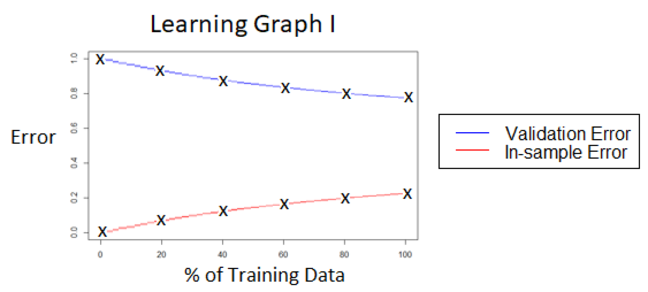There are two important bits of information in a learning graph. Firstly, we have bounds on the maximum possible potential improvement in performance that could occur from adding additional training data. This is given at every point, but is most important at 100%.

Secondly, we get an estimate of how much improvement we would see regarding the generalization error (error on new data) of the model if we were to increase the amount of training data we use. This is the desired marginal value of new data. We also get an estimate of the deterioration in the generalization error rate of the model we should expect if we were to reduce the amount of training data we use.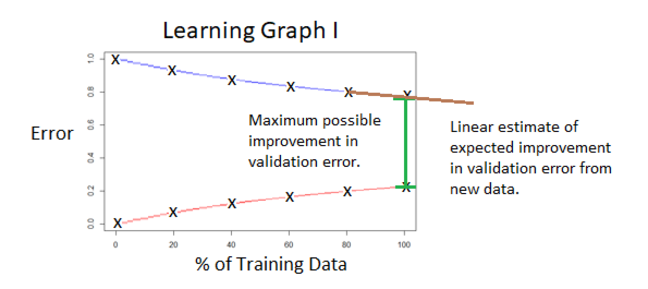This information allows us to make informed decisions about whether we should increase or decrease the amount of training data we are working with. In learning graph I, for example, the marginal value of additional training data is high, and we might wish to add some (presumably taking the added data away from the validation or test data sets). In learning graph II, on the other hand, the marginal value of additional training data is very low, and we might want to remove some training data and use it instead as additional validation or test data.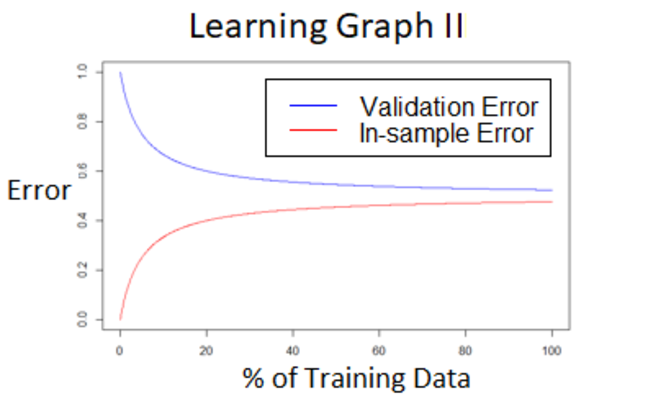While learning graphs can be very useful, they are also difficult to work with. In particular they seldom look as nice and neat as the examples given. Especially with small amounts of data, they can be very noisy, with both curves jumping around markedly, and even regularly crossing each other! (Remember the points calculated are only single value estimates of the expected values of validation and in-sample error of models fitted using the given number of training cases.) In such cases, the learning graphs can typically be massaged into usability by smoothing the estimates, such that instead of plotting the validation and in-sample error rates for each model, you plot the sliding average of $$m$$ such points. See the graphs below for an example of this from real data.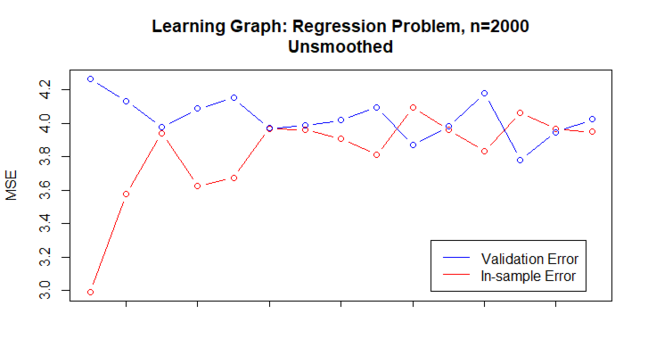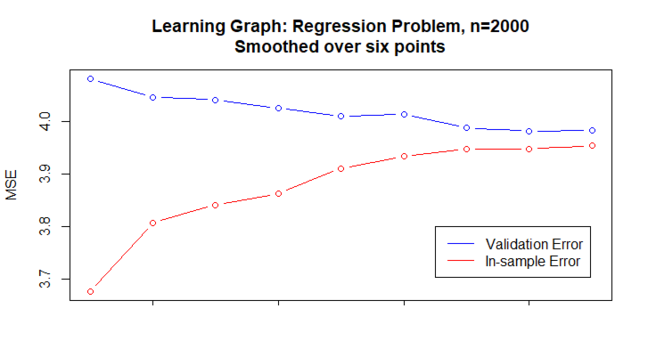## Validation Data: Statistical Hypothesis Tests

Statistical hypothesis tests estimate how confident we should be about rejecting a hypothesis. The hypothesis under consideration for rejection is known as the null hypothesis, $$H_0$$. The hypothesis test will calculate the probability of seeing data ‘as extreme as’ that observed given the null hypothesis. This probability is the p-value of the test. If it is sufficiently low, we can reject the null hypothesis with a high degree of confidence. Typically, the threshold for rejecting the hypothesis is chosen arbitrarily. In the social sciences, values such as .05, .01, and .005. Physics requires p-values below .0000003 before a null hypothesis can be rejected. The key to a hypothesis test is identifying a test statistic that can be calculated from the observed data and which under the null hypothesis is known to come from a calculable probability distribution.

In our case, the hypothesis that we want to reject is that the best and second best models actually have the same expected performance. Accordingly we want to calculate the probability of a difference in performance at least as large as that observed given the two models do have the same expected performance.

The tests that can be used depend upon the type of problem we are working on. We give tests for classification or regression problems here. Note that the statistical hypothesis tests here are implemented in many statistical libraries and these implementations are simple to apply. It is unlikely you will ever need to implement the tests yourself, though for those who are interested, the mathematics for doing so is supplied in asides below the quick introduction of each test.

The significance of differences in error rate between models is very dependent of the size of the data set the error rate is for. With larger data sets almost any difference will be significant.

### Statistical Tests for Classification Tasks

Interested students can get a fuller mathematical explanation of the three statistical hypothesis tests covered here in the Inside the Statistical Hypothesis Tests document available to download at the end of this article.

#### McNemar’s Test

McNemar’s test can be used to evaluate whether the performance of two classifiers, Model A and Model B, are statistically significantly different. To perform this test, we need to be able to complete the contingency table below:

 Model B: Correct Model B: Incorrect Modal A: Correct $$\alpha$$ $$\beta$$ Model A: Incorrect $$\gamma$$ $$\delta$$

Note that we require the number of cases that Model A got correct and Model B got wrong, and vice versa, as well as the number that both got right and both got wrong. In situations where this is not available, the less accurate likelihood ratio test can be used (see below).

#### The Likelihood Ratio Test

The likelihood ratio test can be used to evaluate whether the performance of two classifiers, Model A and Model B, are statistically significantly different based only on their classification (or misclassification) rate. If the additional information required for the McNemar’s test is available, it should be preferred.

### Statistical Tests for Regression Tasks

#### T-Tests

When we are working with regression models, we can use t-tests. The null hypothesis is that the long run error rates of the two models are identical, and the p-value will provide a measure of how probable the observed results were given this hypothesis. To perform a t.test we need a vector for each model giving the error associated with each case in the validation data. If the error rate is (mean) absolute error, this vector will be the absolute value of the residuals (errors). If it is (mean) squared error, then the vector will be the square of the residuals (errors). And so forth.

## Test Data: Confidence Intervals

We use our test data to obtain an unbiased estimate of the chosen model’s performance (using some loss function) on new data. As the amount of test data increases, our confidence that this estimate is close to the true value of the chosen model’s expected performance on new data increases. To evaluate if we have sufficient test data, we need to get some idea of how confident we can be in this estimate given the size of the test data.

Statistical hypothesis tests provide this information, most commonly in the form of confidence intervals. Standard implementations of basic single sample statistical hypothesis tests allow us to request intervals in which we can have some $$n$$ degree of confidence that the true value lies, such as 99.5%. We can examine the size of such confidence intervals and decide whether we are happy about the implied accuracy of our estimate. If not, we will need to increase the amount of test data. If so, we might consider decreasing the amount of test data (though this is difficult to act upon, since we are typically at the end of our experiments at this point).

Regression problems will be able to get confidence intervals around the chosen model’s estimated performance on new data by passing the appropriate error vector to a single sample t-test. The vector will be of the absolute values of the residuals (errors) if working with (mean) absolute error, the squared value of the residuals (errors) if working with (mean) squared error, etc.

Classification models can get confidence intervals from tests like the likelihood ratio test, which requires only the number of successful cases and the total number of cases.